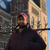Qlik Sense Documents

Qlik Sense documentation and resources.

Announcements
QlikWorld 2022, LIVE in Denver CO., May 16-19, 2022. REGISTER NOW TO RECEIVE EARLY BIRD PRICING

How to Handle Null Count in QlikView and QlikSense?

cancel
Showing results for
Did you mean:Creator III

How to Handle Null Count in QlikView and QlikSense?

NULL is nothing but no value. Null Values will be visible as dashes in QlikView Table Box.

In this article I am going to explain How to handle Null Count in QlikView and Qliksense with different types of Methods using one example for easy understanding.

Find out Nulls in a field is a little bit tricky until you understand the tricks and tips which can make it a little simpler.

For example, I want to calculate the Count of Null Values Present in Value Field.Method 1:

In the above Example, there is Total 17 Null values under Value Field.

To get the Null Count 17 use the below expression. As per this expression when the value is Null and value is equal to Zero then the count will be produced by this expression.

=Sum( IF(ISNULL(Value) OR LEN(trim(Value))=0 OR Value=0,1,0))

To avoid Zero values and to Count only Pure Null Values then need to use the below expression.

=Sum( IF(ISNULL(Value),1,0))

To get non Blank Values then need to use the below expression.

=Sum( IF(ISNULL(Value) OR LEN(trim(Value))=0 OR Value=0,0,1))

Method 2:

Counting Nulls in QlikView and QlikSense is made easy using the NullCount() function which pretty much does exactly as you'd expect. It can be used in both the script and expressions and the syntax is very straightforward:

=NullCount(Value)​​​​​​​

Method 3:

Using MissingCount()

=MissingCount(Value)​​​​​​​

Difference between NullCount() and MissingCount()

The first thing you want to keep in mind is that QlikView and Qlik Sense uses dual values to store all data.

The dual value consists of two components, the value you see in the application and an underlying numerical value.

If you have a value like 1.6, there might be an underlying value that is equal to 1.5875.

If you have a thousand separator the visual value could look like 1,600, while the underlying number is 1600.

When there is no underlying numerical value, the value is considered as a text.

There can never be an underlying numerical value, without a text representing it.

When there is no text, there is no value. This empty space is referred to as a NULL value. NULL is typically presented as a dash in QlikView.

NullCount() will count all values that are defined as NULL.

MissingCount() will count all values without a underlying numerical representation, in other words all NULL values and all texts.

Method 4:

If the value is Null or if the value is like blank/Space (‘ ’) then use the below expression

=Count(if(IsNull(Value)or Value=' ',1))

Method 5:

Using NULLASVALUE() in the Script also we can calculate the Null Count. The NullAsValue statement specifies for which fields (here Value Field) that NULL should be converted to a value.

Use the below code in the back end. Here all the null values will be converted to NULL Text

NULLASVALUE Value;
SET NullValue = 'NULL';
ID,
Value
FROM
(ooxml, embedded labels, table is Sheet1);

In the Front end to see the Null count use the below Expression

= Count({< Value = {'NULL'} >} ID )

Method 6:

In this method we are creating a Flag with True or False. Here 1 represent the Blank records count and 0 represents the Non-Blank records count.

Use the below code in the back end to create a Flag

ID,
Value,
IF(ISNULL(Value) OR LEN(trim(Value))=0 OR Value=0,1,0) as Flag
FROM
(ooxml, embedded labels, table is Sheet1);

In the Front end to see the Null count use the below Expression

=Count({<Flag={'1'}>}Value)Specialist III

An insight for MissingCount() and NullCount()

For examples there are two files in which you have one ID column each, one of the file is Excel and another is csv file.

both the files contains 20 rows but the ID column contains 4 values in both the files , if you write Missing count and NullCount in excel file you will get 16 but if you write in csv you will 16 for Missing count and 0 for Nullcount , so beware of files which don't have concept like Null values, then as kumar told

len() would be appropriate or use SET NULLINTERPRET='', in my example to treat empty cells as Null values

HTH

Kiran KumarChampion

Hi,

one thing concerning Method1: no need to use Isnull() with len(trim())=0

as mentioned here: Use of Len(Trim) with IsNull

len trim will work for NULLS and BLANKS

otherwise Isnull() work for NULLS but not BLANKS

Version history
Last update:
‎2018-07-17 08:49 AM
Updated by: